EqWorld The World of Mathematical EquationsMethods > Partial Differential Equations of Mathematical Physics > The von Mises transformation: order reduction and construction of Bäcklund transformations and new integrable equations

## The von Mises transformation: order reduction and construction of Bäcklund transformations and new integrable equations

Abstract. Wide classes of nonlinear mathematical physics equations are described that admit order reduction through the use of the von Mises transformation, with the unknown function taken as the new independent variable and an appropriate partial derivative taken as the new dependent variable. RF-pairs and associated Bäcklund transformations are constructed for evolution equations of general form (special cases of which are Burgers, Korteweg-de Vries, and Harry Dym type equations as well as many other nonlinear equations of mathematical physics). The results obtained are used for order reduction and constructing exact solutions of hydrodynamics equations. A generalized Calogero equation and a number of other new integrable nonlinear equations are considered.

### 1. Preliminary remarks

A simple analogue of the von Mises transformation is widely used in the theory of ordinary differential equations. Specifically, an nth-order autonomous ordinary differential equation of general form,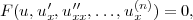(1)

which does not depend explicitly on x, can be reduced with the change of variable(2)

to an (n − 1)st-order equation (e.g., see [1, 2]).

For partial differential equations, the von Mises transformation (with the unknown function and its appropriate partial derivative taken as the new independent and dependent variables, respectively) is applied for order reduction of steady hydrodynamic boundary layer equations [3-5].

An RF-pair is an operation of consecutive raising and lowering of the order of an equation (RF stands for “Rise” and “Fall”). To illustrate this concept, let us solve the nth-order ordinary differential equation of general form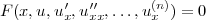(3)

for the independent variable (which is assumed to be feasible) to obtain(4)

Differentiating (4) with respect to x yields an (n + 1)st-order autonomous equation, whose order is further reduced using substitution (2). As a result, we arrive at the nth-order equation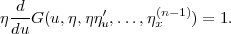(5)

The derivatives with respect to x are found consecutively using ηx(k) = η(ηx(k−1))u. The derivative with respect to u on the left-hand side of (5) is calculated by the chain rule taking into account that F depends on uη, ηu, ...

Equations (4) and (5) are related by the Bäcklund transformation(6)

Many new integrable ordinary differential equations have been found in a systematic manner using RF-pair operations and associated Bäcklund transformations [2, 6].

The above ideas and results for ordinary differential equations turn out to be transferrable, when appropriately modified, to nonlinear partial differential equations. The present paper will describe wide classes of nonlinear mathematical physics equations that admit order reduction with the Mises transformation. This transformation will then be used to construct RF-pairs and Bäcklund transformations for nonlinear evolution equations of general form. This will result in finding new integrable nonlinear equations.

Various Bäcklund transformations and their applications to specific mathematical physics equations can be found, for example, in [5, 7-17].

In the present paper, the term integrable equation applies to nonlinear partial differential equations that admit solution in terms of quadratures or can be linearized (i.e., their solutions can be expressed in terms of solutions to linear differential equations or linear integral equations).

### 2. Using the von Mises transformation for order reduction of partial differential equations

Consider the nonlinear nth-order equation [5, p. 678]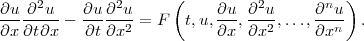(7)

In the special case F = νuxxx, this is a nonlinear third-order equation that describes a laminar boundary layer of a non-Newtonian fluid on a flat plate, with u being the stream function, t and x spatial coordinates measured along and across the plate, and ν the kinematic fluid viscosity [3-5].

1°. Characteristic property of Eq. (7). Let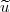(t, x) be a solution to the above equation. Then the functionwith an arbitrary function φ(t), is also a solution to the equation.

2°. In Eq. (7), let us pass from the old variables to the Mises variables:(8)

The derivatives are transformed as follows: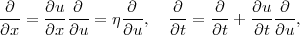(9)(10)

As a result, the von Mises transformation (8) reduces the nth-order equation (7) to the (n − 1)st-order equation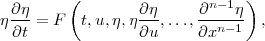(11)

where the higher derivatives are calculated as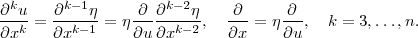Short notation for partial derivatives (e.g., ux, uxx, uxt, etc.) will sometimes be used in what follows.

Example 1. Equation (7) with F = f(t, u)uxx + g(t, u)ux2 + h(t, u)ux = f(t, u)ηηu + g(t, u)η2 + h(t, u)η is reduced to an equation (11) of a special form, which is further reduced, after canceling by η, to a linear first-order equation: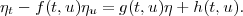Example 2. If F = f(t, u)uxxx/ux = f(t, u)(ηηu)u, Eq. (11) is reduced with the change of variable Z = η2 to a linear second-order equation: Zt = f(t, u)Zuu.

Given below are two possible generalizations of Eq. (7).

1°. Equation (7) can be generalized by including Ix, …, Ix(m), where I = uxutx − utuxx, into F as additional arguments.

2°. The von Mises transformation (8) reduces the order of the equation(12)

and brings it to the form (11), where ηt must be replaced with Gt. If G = ux, Eq. (12) coincides with Eq. (7).

Example 3. The nonlinear third-order equation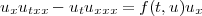(13)

is a special case of Eq. (12) with G = uxx and F = f(t, u)ux. The von Mises transformation (8) brings it to the second-order equation (ηηu)t = f(t, u), whose general solution is given by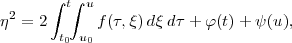where φ(t) and ψ(u) arbitrary functions and t0 and u0 are arbitrary constants.

Remark. Other partial differential equations that admit order reduction will be considered below in Section 6.

### 3. Using the von Mises transformation for constructing RF-pairs and Bäcklund transformations

Consider the nth-order evolution equation of general form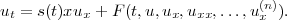(14)

The Burgers, Korteweg-de Vries, and Harry Dym equations as well as many other nonlinear mathematical physics equations [5, 7-9] are special cases of Eq. (14).

1°. Characteristic property of Eq. (14). Let(t, x) be a solution to the above equation. Then the function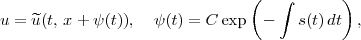where C is an arbitrary constant, is also a solution to this equation.

2°. Suitable RF-pair operations are performed using the formula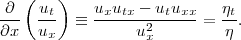(15)

It is obtained by differentiating the ratio of the derivatives ut/ux with respect to x followed by passing from txu to the von Mises variables (8).

Let us divide both sides of Eq. (14) by ux and differentiate with respect to x. The left-hand side of the resulting (n + 1)st-order equation coincides with (15). By passing to the von Mises variables (8) and rearranging, we arrive at the nth-order equation(16)

The partial derivatives with respect to x are calculated successively using ηx(k) = η(ηx(k−1))u. The derivative with respect to u on the right-hand side of Eq. (16) is obtained by the chain rule taking into account that F depends on uη, ηu, …

3°. Equations (14) and (16) are related by the Bäcklund transformation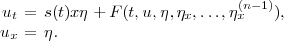(17)

Any solution u = g(t, x) to Eq. (14) determines a solution to Eq. (16), which can be represented in parametric form as u = g(t, x), η = gx(t, x).

Suppose η = η(t, u) is a solution to Eq. (16). Then it follows from the second equation in (17) that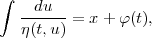(18)

where φ(t) is an arbitrary function. Further, on solving Eq. (18) for u = u(t, u), one should substitute the resulting expression into the original equation (14) and determine φ(t).

Remark. It is sometimes convenient to rewrite Eq. (16) in the form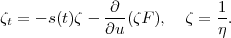### 4. Using the von Mises transformation for constructing new integrable nonlinear equations

Below are examples of using the Bäcklund transformation (17) to construct new integrable nonlinear equations of mathematical physics.

Example 1. Consider the generalized Burgers equation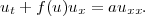(19)

The Bäcklund transformation (17) with F = xf(u)η = aηηuf(u)η and s(t) ≡ 0 brings Eq. (19) to the equation ηt = 2ηuufu(u)η2, which is further reduced, with the substitution η = 1/ζ, to the nonlinear heat equation with a source(20)

By setting f(u) = bu in (19), we arrive at an integrable equation (20) (reducible to the classical Burgers equation (19)), which was derived in  (see also [5, 19]) using a different method.

Example 2. Consider the nonlinear second-order equation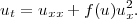(21)

It is reducible, with the change of variable [5, p. 87]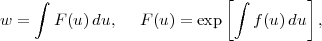to the linear heat equation wt = wxx.

The Bäcklund transformation (17) with F = ηx + f(u)η2 = ηηu + f(u)η2 and s(t) ≡ 0 reduces Eq. (21) to the new integrable nonlinear equation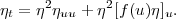Example 3. The linear third-order equation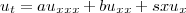with arbitrary functional coefficients a = a(t), b = b(t), and s = s(t) can be reduced, using the Bäcklund transformation (17) with F = xx + x = (ηηu)u + bηηu followed by substituting η = 1/ζ, to a new integrable equation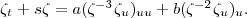(22)

In the special cases a = s = 0 and b = s = 0, we have simpler nonlinear equations, which were studied in  and [5, p. 26, 534].

Example 4. Consider the nonlinear third-order equation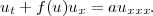(23)

If f(u) ≡ 0, it is linear. For f(u) = bu and f(u) = bu2, it becomes the Korteweg-de Vries and modified Korteweg-de Vries equation [5, 7], respectively. The Bäcklund transformation (17) with F = xxf(u)η = (ηηu)uf(u)η and s(t) ≡ 0 brings Eq. (23) to the equation ηt = aη2(ηηu)uufu(u)η2, which is further reduced with the change of variable η = 1/ζ to the form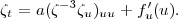(24)

The special case of this equation with f(u) ≡ 0 was obtained in [5, p. 534] by a different method.

Also, setting f(u) = bu and f(u) = bu2 in (24) gives the integrable equations
 ζt = a(ζ−3ζu)uu + b, (25) ζt = a(ζ−3ζu)uu + 2bu. (26)

Example 5. Consider the third-order equation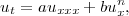(27)

which is linear for n = 0 and n = 1 and also integrable for n = 2 and n = 3 [5, 7]. The Bäcklund transformation (17) with F = auxxx + buxn = (ηηu)u + n and s(t) ≡ 0 brings Eq. (27) to a nonlinear equation, which is further reduced with the change of variable η = θ1/2 to the form(28)

In particular, setting n = 2 and n = 3 in (28) gives the integrable equations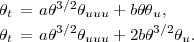Example 6. The nonlinear third-order equation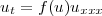(29)

is integrable for f(u) = au3/2 [5, p. 535] (cf. Eq. (28) with b = 0) and f(u) = au3 (Harry Dym equation) [5, p. 528]. The Bäcklund transformation (17) with F = f(u)ηxx = f(u)η(ηηu)u and s(t) ≡ 0 reduces Eq. (29) to the equation ηt = η2[f(u)(ηηu)u]u, which can also be rewritten as

θt = θ3/2[f(u)θuu]u,       θ = η2.

Example 7. The linear fourth-order equation ut = auxxxx can be reduced, using the Bäcklund transformation (17) with F = xxx = [η(ηηu)u]u and s ≡ 0 followed by the change of variable η = θ1/2, to the nonlinear fourth-order equation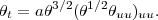(30)

Remark. More new integrable equations may be obtained by applying a Bäcklund transformation of the form (17), based on the von Mises transformation, to each of the equations (20) (with f(u) = bu), (22), (25), (28), and (30).

### 5. Using the von Mises transformation for integro-differential equations

Consider integro-differential equations of the form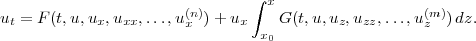(31)

The following brief notation is used for the arguments of G: uz(k) = uz(k)(t, z), k = 0, 1, …, m. In the special case G = s(t) and x0 = 0, Eq. (31) coincides with Eq. (14).

Dividing Eq. (31) by ux, differentiating with respect to x, and passing to the von Mises variables (8), we obtain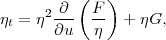(32)

where F = f(t, u, η, ηηu, η(ηηu)u, …) and G = G(t, u, η, ηηu, η(ηηu)u, …).

Equations (31) and (32) are related by the nonlocal Bäcklund transformation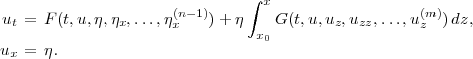(33)

Example. Let us choose the following functions in Eq. (31):(34)

Transformation (33)–(34) leads to the partial differential equationWith the change of variable η = 1/ζ, it is reduced to the linear equation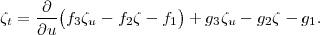Remark. The integro-differential equation (31) can be reduced, by dividing by ux and differentiating with respect to x, to a partial differential equation containing a mixed derivative.

### 6. A new class of nonlinear equations with mixed derivatives that admit order reduction

By setting G = a(t)u in Eq. (31) and substituting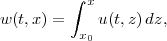(35)

one arrives at the following (n + 1)st-order differential equation with a mixed derivative:(36)

Nonlinear (n + 1)st-order equations of the form (36) can be reduced, using substitution (35) and applying the Bäcklund transformation (33) with G = a(t)u(t, z), to the nth-order equationExample 1 (generalized Calogero equation). The nonlinear second-order equation with a mixed derivative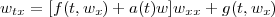(37)

is a special case of Eq. (36) with F = f(t, wx)wxx + g(t, wx). Substitution (35) brings Eq. (37) to an equation of the form (31):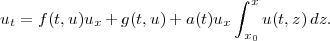(38)

The nonlocal Bäcklund transformation (33) with G = a(t)u(t, z) reduces Eq. (38) to the equationwhich becomes linear after substituting η = 1/ζ.

In the special case of a(t) = 1, f(t, wx) = 0, and g(t, wx) = g(wx), Eq. (37) becomes the Calogero equation [5, 20, 21].

Example 2. Consider the nonlinear third-order equation(39)

which arises in hydrodynamics and derives from the Navier-Stokes equations [5, 22-24]; w is one of the fluid velocity components and ν is the kinematic viscosity. With a(t) = −1, F = νuxx + mu2 + q(t)u + q(t), and u = wx, the above transformations reduce Eq. (39) to a second-order equation representable in the form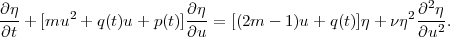(40)

Note that in the degenerate case of inviscid fluid (ν = 0), the original nonlinear equation (39) reduces to a first-order linear equation, Eq. (40) with ν = 0.

### 7. Generalization of the von Mises transformation to the case of three independent variables

The von Mises transformation can be generalized to the cases of three or more independent variables. For example, let us consider the nth-order equation with three independent variables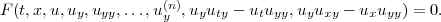(41)

The von Mises transformation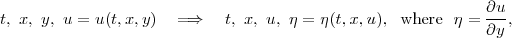(42)

reduces the order of Eq. (41) and brings it to the form(43)

where the y-derivatives are calculated as ηy(k) = η(ηy(k−1))u.

Example. Consider the Prandtl system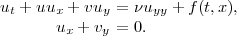(44)

It describes a plane unsteady boundary layer with pressure gradient; u and v are the fluid velocity components [3-5]. Let us divide the first equation by uy, then differentiate with respect to y, and eliminate vy using the second equation in (44) to obtain a nonlinear third-order equation of the form (41):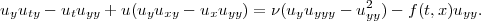(45)

Applying the von Mises transformation (42) to Eq. (45) yields the second-order equation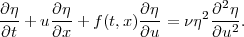(46)

Once a solution to this equation has been found, the second velocity component v is found immediately from the first equation of (44) without integrating.

Note that Eq. (46) can be reduced, with the change of variable η = 1/ζ, to the nonlinear heat equation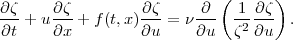(47)

1°. Consider the special case f(t, x) = f(t). We look for exact solutions to Eq. (47) in the form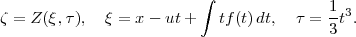We arrive at the integrable equation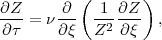(48)

which can be reduced to the linear heat equation  (see also ).

2°. Consider the more general case f(t, x) = f(t)x + g(t). We look for exact solutions of the special form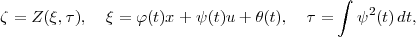where φ = φ(t), ψ = ψ(t), and θ = θ(t) are determined by the linear system of ordinary differential equations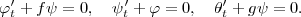As a result, we arrive at the integrable equation (48).

### Conclusion

We have presented wide classes of nonlinear mathematical physics equations that admit order reduction through the use of the von Mises transformation, with the unknown function taken as the new independent variable and an appropriate partial derivative taken as the new dependent variable. RF-pairs and associated Bäcklund transformations are constructed for evolution equations of general form (special cases of which are Burgers and Korteweg-de Vries type equations as well as many other nonlinear equations of mathematical physics). The results obtained are used for order reduction and constructing exact solutions of hydrodynamics equations. A generalized Calogero equation and a number of other new integrable nonlinear equations are considered.

The von Mises and Bäcklund transformations described in the paper can be used to construct other new integrable nonlinear differential and integro-differential equations of mathematical physics.

### Acknowledgments

The work was carried out under partial financial support of the Russian Foundation for Basic Research (grants No. 08-01-00553, No. 08-08-00530 and No. 09-01-00343).

### References

 Murphy G. M. Ordinary Differential Equations and Their Solutions. D. Van Nostrand, New York, 1960.

 Polyanin A. D., Zaitsev V. F. Handbook of Exact Solutions for Ordinary Differential Equations, 2nd Edition. Chapman & Hall/CRC Press, Boca Raton, 2003.

 Loitsyanskiy L. G. Mechanics of Liquids and Gases. Begell House, New York, 1996.

 Schlichting H. Boundary Layer Theory. McGraw-Hill, New York, 1981.

 Polyanin A. D., Zaitsev V. F. Handbook of Nonlinear Partial Differential Equations. Chapman & Hall/CRC Press, Boca Raton, 2004.

 Zaitsev V. F., Polyanin A. D. Discrete-Group Methods for Integrating Equations of Nonlinear Mechanics. Begell House/CRC Press, Boca Raton, 1994.

 Ibragimov N. H. CRC Handbook of Lie Group Analysis of Differential Equations, Vols. 1-2. CRC Press, Boca Raton, 1994-1995.

 Bäcklund Transformations. Ed. by R. M. Miura. Springer-Verlag, Berlin, 1975.

 Rogers C., Shadwick W. F. Bäcklund Transformations and Their Applications. Academic Press, New York, 1982.

 Nimmo J. J. C., Crighton D. J. Bäcklund transformations for nonlinear parabolic equations: the general results. Proc. Royal Soc. London A, 1982, Vol. 384, pp. 381-401.

 Kingston J. G., Rogers C., Woodall D. Reciprocal auto-Bäcklund transformations. J. Physics A: Math. Gen., 1984, Vol. 17, No. 2, pp. L35-L38.

 Weiss J. Bäcklund transformations and the Painlevé property. J. Math. Phys., 1986, Vol. 27, No. 5, pp. 1293-1305.

 Nucci M. C. Nonclassical symmetries and Bäcklund transformations. J. Math. Anal. Appl., 1993, Vol. 178, pp. 294-300.

 Fordy A. P. A historical introduction to solitons and Bäcklund transformations. In: A.P. Fordy and J.C. Wood, Eds, Harmonic Maps and Integrable Systems, pp. 7-28. Vieweg, Wiesbaden, 1994.

 Hong W. P. On Bäcklund transformation for a generalized Burgers equation and solitonic solutions. Physics Letters A, 2000, Vol. 268, No. 1-2, pp. 81-84.

 Wang M., Wang Y., Zhou Y. An auto-Bäcklund transformation and exact solutions to a generalized KdV equation with variable coefficients and their applications. Physics Letters A, 2002, Vol. 303, No. 1, pp. 45-51.

 Yonga X., Zhangb Z., Chen Y. Bäcklund transformation, nonlinear superposition formula and solutions of the Calogero equation. Physics Letters A, 2008, Vol. 372, No. 41, pp. 6273-6279.

 Dorodnitsyn V. A., Svirshchevskii S. R. On Lie-Bäcklund Groups Admitted by the Heat Equation with a Source [in Russian]. Preprint No. 101, Keldysh Institute of Applied Mathematics, U.S.S.R. Academy of Sciences, Moscow, 1983.

 Bluman G. W., Kumei S. On the remarkable nonlinear diffusion equation [a(u + b)−2ux]xut = 0. J. Math. Phys., 1980, Vol. 21, No. 5, pp. 1019-1023.

 Calogero F. A solvable nonlinear wave equation. Stud. Appl. Math., 1984, Vol. 70, No. 3, pp. 189-199.

 Pavlov M. V. The Calogero equation and Liouville-type equations, Theor. & Math. Phys., 2001, Vol. 128, No. 1, pp. 927-932.

 Polyanin A. D. Exact solutions to the Navier-Stokes equations with generalized separation of variables. Doklady Physics, 2001, Vol. 46, No. 10, pp. 726-731.

 Pukhnachev V. V. Symmetries in the Navier-Stokes equations [in Russian]. Uspekhi Mekhaniki [Advances in Mechanics], 2006, Vol. 4, No. 1, pp. 6-76.

 Aristov S. N., Polyanin A. D. Exact solutions of unsteady three-dimensional Navier-Stokes equations. Doklady Physics, 2009, Vol. 54, No. 7, pp. 316-321.

A.D. Polyanin and A.I. Zhurov, The von Mises transformation: order reduction and construction of Bäcklund transformations and new integrable equations, 3 July 2009. Website EqWorld — The World of Mathematical Equations, http://eqworld.ipmnet.ru/en/methods/methods-pde/mises/mises-transformation.htm